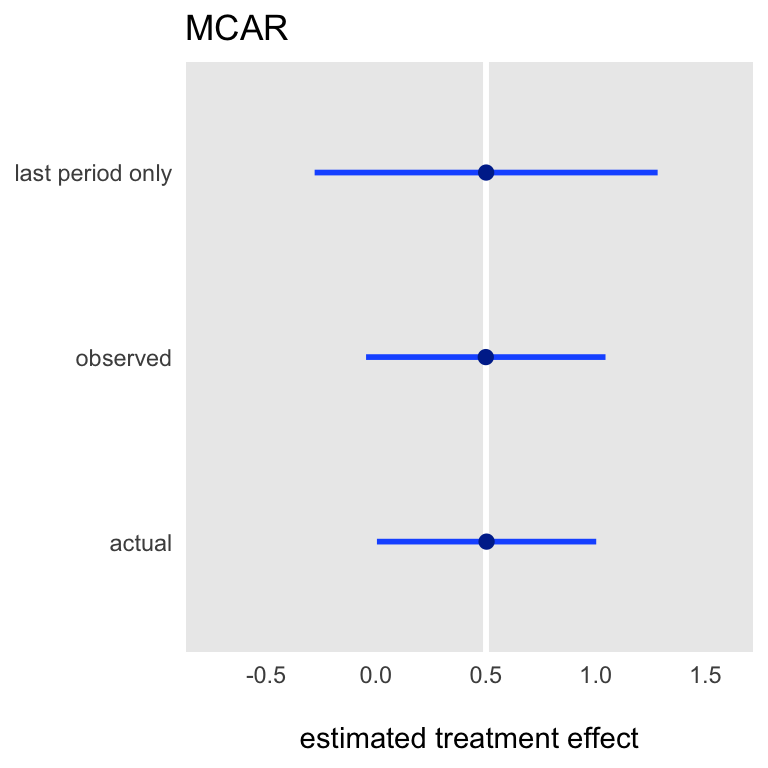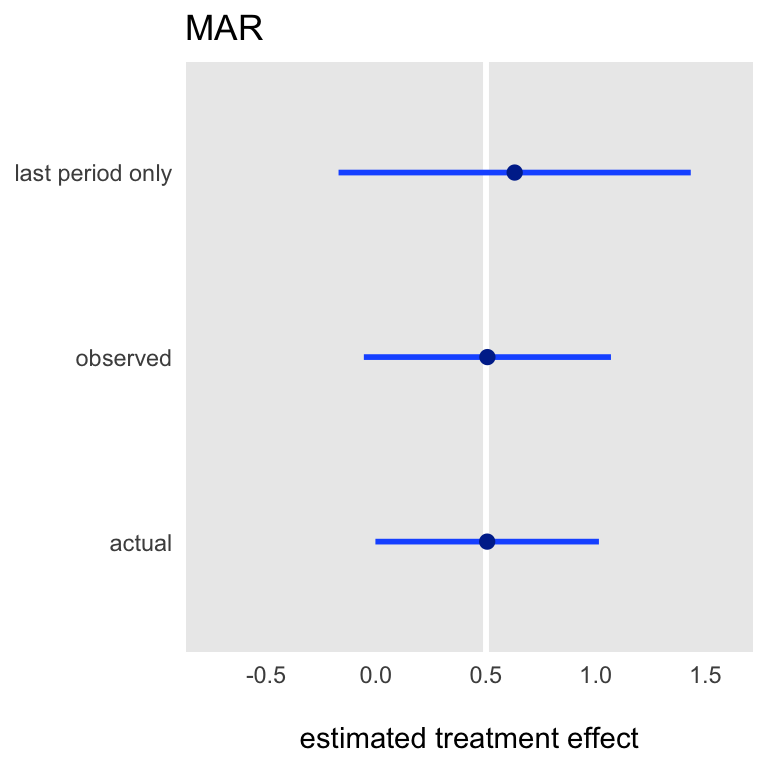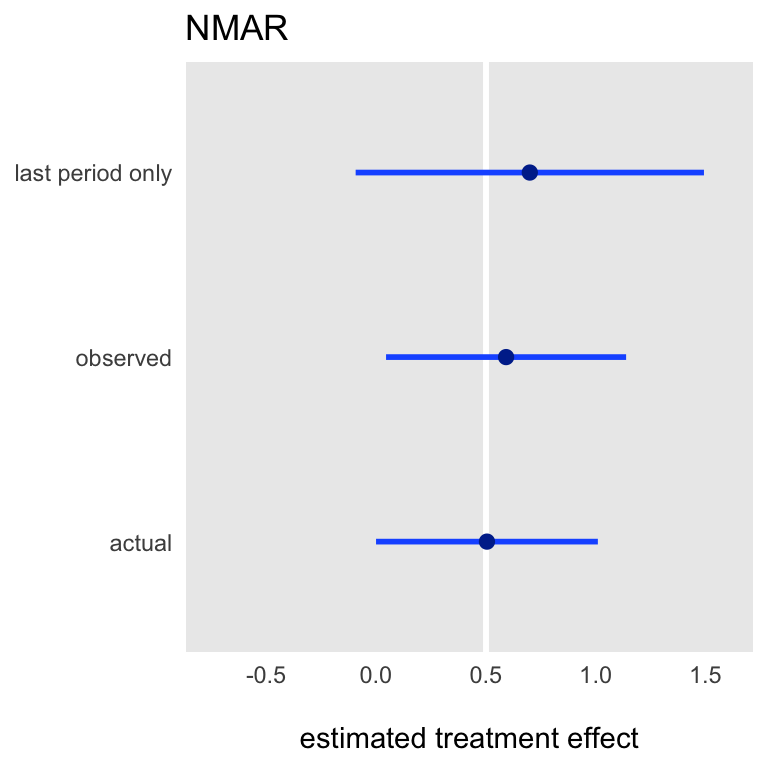# Repeated measures can improve estimation when we only care about a single endpoint

I’m participating in the design of a new study that will evaluate interventions aimed at reducing both pain and opioid use for patients on dialysis. This study is likely to be somewhat complicated, possibly involving multiple clusters, multiple interventions, a sequential and/or adaptive randomization scheme, and a composite binary outcome. I’m not going into any of that here.

There is one issue that should be fairly generalizable to other studies. It is likely that individual measures will be collected repeatedly over time but the primary outcome of interest will be the measure collected during the last follow-up period. I wanted to explore what, if anything, can be gained by analyzing all of the available data rather than focusing only the final end point.

### Data generation

In this simulation scenario, there will be 200 subjects randomized at the individual level to one of two treatment arms, intervention ($$rx = 1$$) and control ($$rx = 0$$). Each person will be followed for 5 months, with a binary outcome measure collected at the end of each month. In the data, period 0 is the first month, and period 4 is the final month.

library(simstudy)

set.seed(281726)

dx <- genData(200)
dx <- trtAssign(dx, grpName = "rx")
dx <- addPeriods(dx, nPeriods = 5)

Here are the data for a single individual:

dx[id == 142]
##     id period rx timeID
## 1: 142      0  1    706
## 2: 142      1  1    707
## 3: 142      2  1    708
## 4: 142      3  1    709
## 5: 142      4  1    710

The probabilities of the five binary outcomes for each individual are a function of time and intervention status.

defP <- defDataAdd(varname = "p",
formula = "-2 + 0.2*period + 0.5*rx",
dist = "nonrandom", link = "logit")

dx <- addColumns(defP, dx)

The outcomes for a particular individual are correlated, with outcomes in two adjacent periods are more highly correlated than outcomes collected further apart. (I use an auto-regressive correlation structure to generate these data.)

dx <- addCorGen(dtOld = dx, idvar = "id", nvars = 5, rho = 0.6,
corstr = "ar1", dist = "binary", param1 = "p",
method = "ep", formSpec = "-2 + 0.2*period + 0.5*rx",
cnames = "y")

dx[id == 142]
##     id period rx timeID    p y
## 1: 142      0  1    706 0.18 0
## 2: 142      1  1    707 0.21 0
## 3: 142      2  1    708 0.25 1
## 4: 142      3  1    709 0.29 0
## 5: 142      4  1    710 0.33 0

In the real world, there will be loss to follow up - not everyone will be observed until the end. In the first case, I will be assuming the data are missing completely at random (MCAR), where missingness is independent of all observed and unobserved variables. (I have mused on missingess before.)

MCAR <- defMiss(varname = "y", formula = "-2.6",
logit.link = TRUE, monotonic = TRUE
)

dm <- genMiss(dx, MCAR, "id", repeated = TRUE, periodvar = "period")
dObs <- genObs(dx, dm, idvars = "id")

dObs[id == 142]
##     id period rx timeID    p  y
## 1: 142      0  1    706 0.18  0
## 2: 142      1  1    707 0.21  0
## 3: 142      2  1    708 0.25  1
## 4: 142      3  1    709 0.29 NA
## 5: 142      4  1    710 0.33 NA

In this data set only about 70% of the total sample is observed - though by chance there is different dropout for each of the treatment arms:

dObs[period == 4, .(prop.missing = mean(is.na(y))), keyby = rx]
##    rx prop.missing
## 1:  0         0.28
## 2:  1         0.38

### Estimating the intervention effect

If we are really only interested in the probability of a successful outcome in the final period, we could go ahead and estimate the treatment effect using a simple logistic regression using individuals who were available at the end of the study. The true value is 0.5 (on the logistic scale), and the estimate here is close to 1.0 with a standard error just under 0.4:

fit.l <- glm(y ~ rx, data = dObs[period == 4], family = binomial)
coef(summary(fit.l))
##             Estimate Std. Error z value Pr(>|z|)
## (Intercept)    -1.25       0.28    -4.4  9.9e-06
## rx              0.99       0.38     2.6  9.3e-03

But, can we do better? Fitting a longitudinal model might provide a more stable and possibly less biased estimate, particularly if the specified model is the correct one. In this case, I suspect it will be an improvement, since the data was generated using a process that is amenable to a GEE (generalized estimating equation) model.

library(geepack)

fit.m <- geeglm(y ~ period + rx, id = id, family = binomial,
data = dObs, corstr = "ar1")

coef(summary(fit.m))
##             Estimate Std.err Wald Pr(>|W|)
## (Intercept)    -2.33   0.259   81  0.00000
## period          0.30   0.072   17  0.00003
## rx              0.83   0.263   10  0.00152

And finally, it is reasonable to expect that a model that is based on a data set without any missing values would provide the most efficient estimate. And that does seem to be case if we look at the standard error of the effect estimate.

fit.f <- geeglm(y ~ period + rx, id = id, family = binomial,
data = dx, corstr = "ar1")

coef(summary(fit.f))
##             Estimate Std.err Wald Pr(>|W|)
## (Intercept)    -2.15   0.227 89.2  0.0e+00
## period          0.30   0.062 23.1  1.5e-06
## rx              0.54   0.233  5.4  2.1e-02

Of course, we can’t really learn much of anything from a single simulated data set. Below is a plot of the mean estimate under each modeling scenario (along with the blue line that represents $$\pm 2$$ sd) based on 2500 simulated data sets with missingness completely at random. (The code for these replications is included in the addendum.)

It is readily apparent that under an assumption of MCAR, all estimation models yield unbiased estimates (the true effect size is 0.5), though using the last period only is inherently more variable (given that there are fewer observations to work with).### Missing at random

When the data are MAR (missing at random), using the last period only no longer provides an unbiased estimate of the effect size. In this case, the probability of missingness is a function of time, intervention status, and the outcome from the prior period, all of which are observed. This is how I’ve defined the MAR process:

MAR <- defMiss(varname = "y",
formula = "-2.9 + 0.2*period - 2*rx*LAG(y)",
logit.link = TRUE, monotonic = TRUE
)

The mean plots based on 2500 iterations reveal the bias of the last period only. It is interesting to see that the GEE model is not biased, because we have captured all of the relevant covariates in the model. (It is well known that a likelihood method can yield unbiased estimates in the case of MAR, and while GEE is not technically a likelihood, it is a quasi-likelihood method.)### Missing not at random

When missingness depends on unobserved data, such as the outcome itself, then GEE estimates are also biased. For the last set of simulations, I defined missingness of $$y$$ in any particular time period to be a function of itself. Specifically, if the outcome was successful and the subject was in the intervention, the subject would be more likely to be observed:

NMAR <- defMiss(varname = "y",
formula = "-2.9 + 0.2*period - 2*rx*y",
logit.link = TRUE, monotonic = TRUE
)

Under the assumption of missingness not at random (NMAR), both estimation approaches based on the observed data set with missing values yields an biased estimate, though using all of the data appears to reduce the bias somewhat:iter <- function(n, np, defM) {

dx <- genData(n)
dx <- trtAssign(dx, grpName = "rx")
dx <- addPeriods(dx, nPeriods = np)

defP <- defDataAdd(varname = "p", formula = "-2 + 0.2*period + .5*rx",
dist = "nonrandom", link = "logit")

dx <- addCorGen(dtOld = dx, idvar = "id", nvars = np, rho = .6,
corstr = "ar1", dist = "binary", param1 = "p",
method = "ep", formSpec = "-2 + 0.2*period + .5*rx",
cnames = "y")

dm <- genMiss(dx, defM, "id", repeated = TRUE, periodvar = "period")
dObs <- genObs(dx, dm, idvars = "id")

fit.f <- geeglm(y ~ period + rx, id = id, family = binomial,
data = dx, corstr = "ar1")

fit.m <- geeglm(y ~ period + rx, id = id, family = binomial,
data = dObs, corstr = "ar1")

fit.l <- glm(y ~ rx, data = dObs[period == (np - 1)], family = binomial)

return(data.table(full = coef(fit.f)["rx"],
miss = coef(fit.m)["rx"],
last = coef(fit.l)["rx"])
)
}

## defM

MCAR <- defMiss(varname = "y", formula = "-2.6",
logit.link = TRUE, monotonic = TRUE
)

MAR <- defMiss(varname = "y",
formula = "-2.9 + 0.2*period - 2*rx*LAG(y)",
logit.link = TRUE, monotonic = TRUE
)

NMAR <- defMiss(varname = "y",
formula = "-2.9 + 0.2*period - 2*rx*y",
logit.link = TRUE, monotonic = TRUE
)

##

library(parallel)

niter <- 2500

resMCAR <- rbindlist(mclapply(1:niter, function(x) iter(200, 5, MCAR)))
resMAR <- rbindlist(mclapply(1:niter, function(x) iter(200, 5, MAR)))
resNMAR <- rbindlist(mclapply(1:niter, function(x) iter(200, 5, NMAR)))